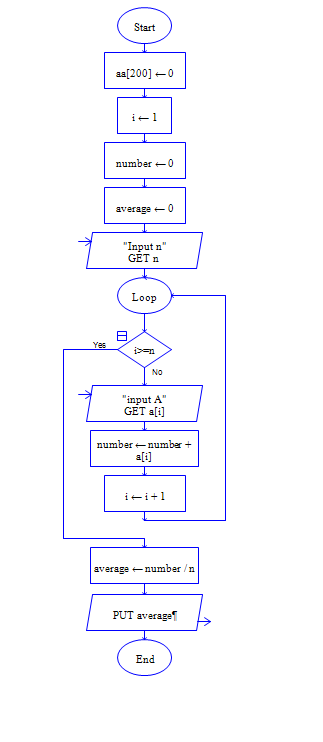# Java Program to find the average of array Elements

Get array size n and n elements of array, then compute average of the elements.

Sample Input 1:

5 5 7 9 3 1

Sample Output 1:

25 5.0

#### Flow Chart Design#### Program or Solution

``` import java.util.*; class SAvArray { public static void main(String args[]) { int size,i,sum=0; double avg; Scanner sc=new Scanner(System.in); System.out.println("Enter Size Of Array:"); size=sc.nextInt(); int a[]=new int[size]; System.out.println("Enter The Array Elements:\n"); for(i=0;i<size;i++) { a[i]=sc.nextInt(); sum=sum+a[i]; } System.out.println("The Sum Of Array Elements Is:\n"+sum); avg=sum/size; System.out.println("The Average Is:"+avg); } } ```

#### Program Explanation

Array is a Collection of data with same type.

1. Get the size of the Array

2. Create a array with the given size (Array has 0 to size-1 index to access every location)

0                     1                        2                       3                  ...........             size-2                  size-1

for(i=0;i<size;i++)

Here i starts at 0, incremented by 1 at every iteration and finally iteration stops when i is equal to size.

Therefore,

In First iteration i is 0, so a[i] is a

In second iteration i is 1, so a[i] is a

....

In last iteration i is size-1, so a[i] is a[size-1]

the input statement nextInt() reads input and stores in array location consecutively. like a, a, a.....

The second For Loop

sums the values of array consecutively like

sum = sum + a[i]

sum = sum + a in first iteration

sum = sum + a in second iteration

......

sum = sum + a[size-1] in last iteration

Finally divide sum by size to find the average.

print average.